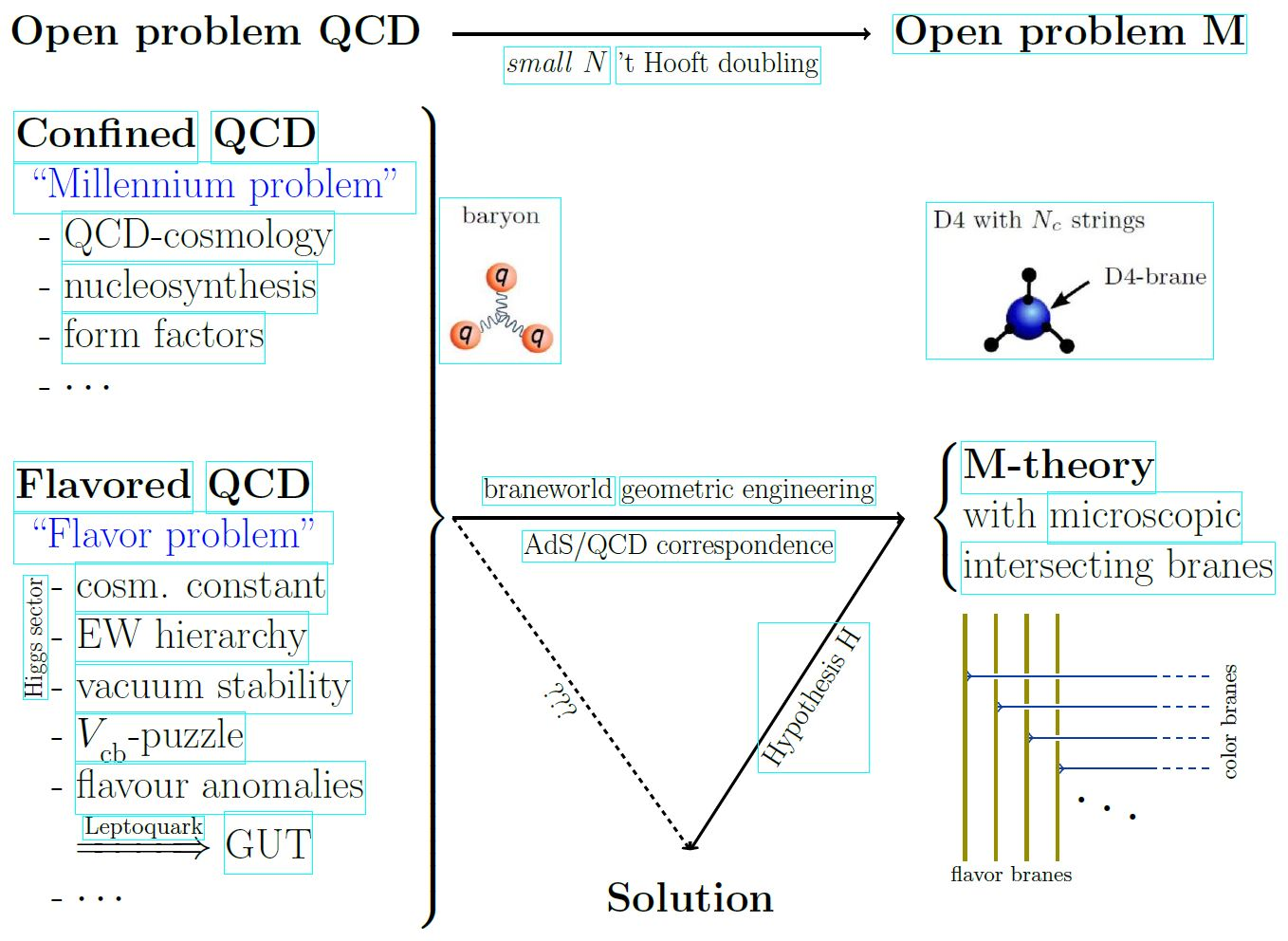Contents

# Contents

## Idea

In the context of the 't Hooft double line construction and the AdS/CFT correspondence with $N_c \in \mathbb{N}$ colors, the small $N$ region or large $1/N_c$ limit is the situation away from the large N limit. In this large $1/N_c$ limit perturbative string theory-corrections (for small 't Hooft coupling $\lambda = g_{YM}^2 N_c$) and/or M-theory-corrections (for small $N_c$) to the supergravity-approximation of the AdS/CFT correspondence are relevant.

In application to phenomenology, the small $N_c$ region (and hence M-theory) is what is ultimately relevant for the AdS/QCD correspondence, given that quantum chromodynamics has a small number $N_c^{QCD} = 3$ of quark colors:### General

On the general relevance of M-theory at small $N_c$ in gauge/gravity duality:

On the logical equivalence between the four-colour theorem and a statement about transition from the small N limit to the large N limit for Lie algebra weight systems on Jacobi diagrams via the 't Hooft double line construction:

#### General

• B. Basso, Cusp anomalous dimension in planar maximally supersymmetric Yang-Mills theory (spire:858223)

“The result $[$(29)$]$ coincides exactly with the recent two-loop stringy correction computed in Alday-Maldacena 07, providing a striking confirmation of the AdS/CFT correspondence.”

• David Jorrin, Nicolas Kovensky, Martin Schvellinger, Towards $1/N$ corrections to deep inelastic scattering from the gauge/gravity duality, JHEP 04 (2016) 113 (arXiv:1601.01627)

Discussion of small N corrections via a lattice QFT-Ansatz on the AdS side of AdS2/CFT1:

• Richard C. Brower, Cameron V. Cogburn, A. Liam Fitzpatrick, Dean Howarth, Chung-I Tan, Lattice Setup for Quantum Field Theory in $AdS_2$ (arXiv:1912.07606)

#### Via conformal bootstrap

Using the conformal bootstrap for CFTs at small N to deduce M-theory-properties on the dual side:

Specifically for the D=6 N=(2,0) SCFT on the M5-brane via AdS7/CFT6:

Specifically for the D=3 SCFT (BLG-model, ABJM model) on the M2-brane via AdS4/CFT3

Specifically in AdS5/CFT4 via D3-brane contributions:

• Yosuke Imamura, Finite-$N$ superconformal index via the AdS/CFT correspondence (arXiv:2108.12090)